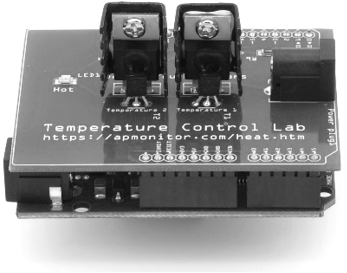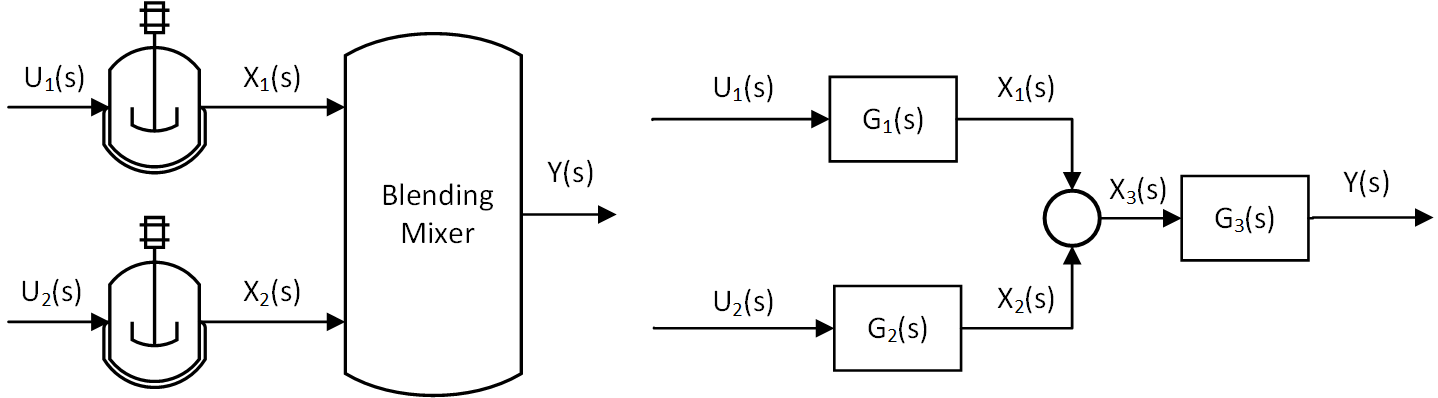## Quiz on Transfer FunctionsInformation for Questions 1-3: Two reactors are operated in parallel and the output of each is mixed in a blending tank. The inputs (U_1 and U_2) are cooling jacket temperatures. The reactor outputs (X_1 and X_2) are reactor concentrations that are mixed (X_3) and blended (Y).1. What is the expression for reactor outlet X_2 in terms of input U_2?

A. X_2 = X_1 U_1
Incorrect. Use the transfer function G_2 and input U_2
B. X_2 = G_1 U_2
Incorrect. Use the transfer function G_2
C. X_2 = G_2 U_2
Correct. The transfer function G_2 relates the input U_2 to the output X_2
D. X_2 = G_1 U_1
Incorrect. Use the transfer function G_2 and input U_2

2. What is the expression for the mixed reactor outlet X_3 in terms of inputs U_1 and U_2?

A. X_3 = G_1 G_2 U_1 U_2
Incorrect. Processes in parallel are additive, not multiplicative
B. X_3 = X_1 U_1 + X_2 U_2
Incorrect. Use transfer functions G_1 and G_2 instead of X_1 and X_2
C. X_3 = X_1 + X_2
Incorrect. This is correct but it is not in terms of the inputs
D. X_3 = G_1 U_1 + G_2 U_2
Correct. X_3 = X_1 + X_2 = G_1 U_1 + G_2 U_2

3. What is the expression for the blender outlet Y in terms of inputs U_1 and U_2?

A. Y = G_3 X_3
Incorrect. This is correct but not in terms of U_1 and U_2
B. Y = G_3 (X_1 + X_2)
Incorrect. This is correct but not in terms of U_1 and U_2
C. Y = G_3 G_1 U_1 + G_3 G_2 U_2
Correct. This is in terms of the inputs U_1 and U_2 and the transfer functions
D. Y = G_3 X_3 G_1 X_1 U_1 + G_3 X_3 G_2 X_2 U_2
Incorrect. The intermediate signals X_1, X_2, and X_3 are not in the input to output expression

4. What are the requirements to obtain a transfer function? Select two correct answers.

A. Nonlinear equations
Incorrect. Only linear equations or linearized nonlinear equations can be transformed to Laplace domain
B. Linear equations
Correct. Only linear equations can be transformed to Laplace domain
C. Differential equation with multiple inputs and one output
Incorrect. A transfer function relates one input to one output
D. Differential equation with one input and one output
Correct. A transfer function relates one input to one output

5. What is the gain of the transfer function G(s) = {5(s + 1)}/{(s + 3)^2}?

A. 5/3
Incorrect. K_p = \lim_{s \to 0} {5(s + 1)}/{(s + 3)^2}
B. 5/9
Correct. K_p = \lim_{s \to 0} {5(s + 1)}/{(s + 3)^2} = {5(1)}/{3^2} = 5/9
C. 0
Incorrect. K_p = \lim_{s \to 0} {5(s + 1)}/{(s + 3)^2}
D. 1
Incorrect. K_p = \lim_{s \to 0} {5(s + 1)}/{(s + 3)^2}